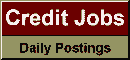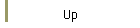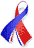DefaultRisk.com the web's biggest credit risk modeling resource.doi> search: A or B Cited by these papersRelated articlesAlternative sources Search RePEc Export citation to:- HTML- Text (plain)- BibTeX- RIS- ReDIF

Finite Maturity Optimal Stopping of Levy Processes with Running Cost, Stopping Cost and Terminal Gain

by Budhi Arta Surya of Bandung Institute of Technology

July 30, 2012

Abstract: Motivated by problems in mathematical finance and insurance, this paper discusses optimal stopping problem in general setting. It considers discounted running cost and stopping cost in addition to terminal gain in the objective function, subject to be optimized over finite-time period. The underlying source of uncertainty is modeled by Lévy processes.We derive early exercise premium representation for the value function based on a partial integro-differential free-boundary problem associated with the optimal stopping problem. The representation gives rise to a nonlinear integral equation for the optimal stopping boundary. The integral equation generalizes that of found in Kim , Myneni , Carr et al. , Jacka , Pham , and Peskir . The boundary can be characterized as a unique solution of the integral equation within the class of continuous decreasing function of time to maturity. We show that the continuity of the boundary holds when the stopping cost function is either time-independent or decreasing in time. Uniqueness of such solution holds when the running cost and stopping cost functions satisfy a differential inequality. By reformulating the free-boundary problem as a linear complementarity, the problem is solved iteratively by adapting the implicit-explicit method of Cont and Voltchkova  and the Brennan-Schwartz  algorithm that was implemented in Jaillet et al.  and Almendral  for the pricing of American put option.We give an example in optimal capital structure. We also verify numerically the recent results in Kyprianou and Surya  that the smooth pasting condition may not hold for general Lévy processes.

Keywords: Optimal stopping, free-boundary, linear complementarity problem.

Books Referenced in this paper:  (what is this?)